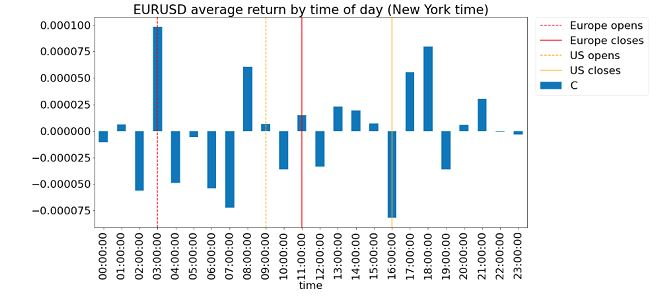## Jumat, 05 September 2014

### Moving Average Crossover = Triangle Filter on 1-Period Returns

Many traders who use technical analysis favor the Moving Average Crossover as a momentum indicator. They compute the short-term minus the long-term moving averages of prices, and go long if this indicator just turns positive, or go short if it turns negative. This seems intuitive enough. What isn't obvious, however, is that MA Crossover is nothing more than an estimate of the recent average compound return.

But just when you might be tempted to ditch this indicator in favor of the average compound return, it can be shown that the MA Crossover is also a triangle filter on the 1-period returns. (A triangle filter in signal processing is a set of weights imposed on a time series that increases linearly with time up to some point, and then decreases linearly with time up to the present time. See the diagram at the end of this article.) Why is this interpretation interesting? That's because it leads us to consider other, more sophisticated filters (such as the least square, Kalman, or wavelet filters) as possible momentum indicators. In collaboration with my former workshop participant Alex W. who was inspired by this paper by Bruder et. al., we present the derivations below.

===

First, note that we will compute the moving average of log prices y, not raw prices. There is of course no loss or gain in information going from prices to log prices, but it will make our analysis possible. (The exact time of the crossover, though, will depend on whether we use prices or log prices.) If we write MA(t, n1) to denote the moving average of n1 log prices ending at time t, then the moving average crossover is MA(t, n1)-MA(t, n2), assuming n1< n2.  By definition,

MA(t, n1)=(y(t)+y(t-1)+...+y(t-n1+1))/n1

MA(t, n2)=(y(t)+y(t-1)+...+y(t-n1+1)+y(t-n1)+...+y(t-n2+1)/n2

MA(t, n1)-MA(t, n2)

=[(n2-n1)/(n1*n2)] *[y(t)+y(t-1)+...+y(t-n1+1)] - (1/n2)*[y(t-n1)+...+y(t-n2+1)]

=[(n2-n1)/n2] *MA(t, n1)-[(n2-n1)/n2]*MA(t-n1, n2-n1)

=[(n2-n1)/n2]*[MA(t, n1)-MA(t-n1, n2-n1)]

If we interpret MA(t, n1) as an approximation of the log price at the midpoint (n1-1)/2 of the time interval [t-n1+1, t], and MA(t-n1, n2-n1) as an approximation of the log price at the midpoint (n2-n1-1)/2 of the time interval [t-n1, t-(n2-n1)], then [MA(t, n1)-MA(t-n1, n2-n1)] is an approximation of the total return over a time period of n2/2. If we write this total return as an average compound growth rate r multiplied by the period n2/2, we get

MA(t, n1)-MA(t, n2)  ≈ [(n2-n1)/n2]*(n2/2)*r

r ≈ [2/(n2-n1)]*[MA(t, n1)-MA(t, n2)]

as shown in Equation 4 of the paper cited above. (Note the roles of n1 and n2 are reversed in that paper.)

===

Next, we will show why the MA crossover is also a triangle filter on 1-period returns. Simplifying notation by fixing t to be 0,

MA(t=0, n1)

=(y(0)+y(-1)+...+y(-n1+1))/n1

=(1/n1)*[(y(0)-y(-1))+2(y(-1)-y(-2))+...+n1*(y(-n1+1)-y(-n1))]+y(-n1)

Writing the returns from t-1 to t as R(t), this becomes

MA(t=0, n1)=(1/n1)*[R(0)+2*R(-1)+...+n1*R(-n1+1)]+y(-n1)

Similarly,

MA(t=0, n2)=(1/n2)*[R(0)+2*R(-1)+...+n2*R(-n2+1)]+y(-n2)

So MA(0, n1)-MA(0, n2)

=(1/n1-1/n2)*[R(0)+2*R(-1)+...+n1*R(-n1+1)]

-(1/n2)*[(n1+1)*R(-n1)+(n1+2)*R(-n1-1)+...+n2*R(-n2+1)]

+y(-n1)-y(-n2)

Note that the last line above is just the total cumulative return from -n2 to -n1, which can be written as

y(-n1)-y(-n2)=R(-n1)+R(-n1-1)+...+R(-n2+1)

Hence we can absorb that into the expression prior to that

MA(0, n1)-MA(0, n2)

=(1/n1-1/n2)*[R(0)+2*R(-1)+...+n1*R(-n1+1)]

-(1/n2)*[(n1+1-n2)*R(-n1)+(n1+2-n2)*R(-n1-1)+...+(-1)*R(-n2+2)]

=(1/n1-1/n2)*[R(0)+2*R(-1)+...+n1*R(-n1+1)]

+(1/n2)*[(n2-n1-1)*R(-n1)+(n2-n1-2)*R(-n1-1)+...+R(-n2+2)]

We can see the coefficients of R's from t=-n2+2 to -n1 form the left side of an triangle with positive slope, and those from  t=-n1+1 to 0 form the right side of the triangle with negative slope. The plot (click to enlarge) below shows the coefficients as a function of time, with n2=10, n1=7, and current time as t=0. The right-most point is the weight for R(0): the return from t=-1 to 0.

Q.E.D. Now I hope you are ready to move on to a wavelet filter!

P.S. It is wonderful to be able to check the correctness of messy algebra like those above with a simple Matlab program!

===

New Service Announcement

Our firm QTS Capital Management has recently launched a FX Managed Accounts program. It uses one of the mean-reverting strategies we have been trading successfully in our fund for the last three years, and is still going strong despite the low volatility in the markets. The benefits of a managed account are that clients retain full ownership and control of their funds at all times, and they can decide what level of leverage they are comfortable with. Unlike certain offshore FX operators, QTS is a CPO/CTA regulated by the National Futures Association and the Commodity Futures Trading Commission.

===

Workshops Update

===